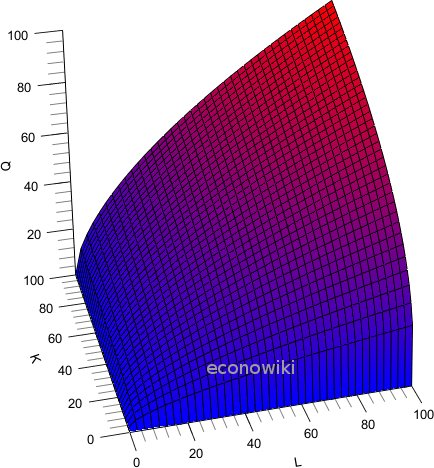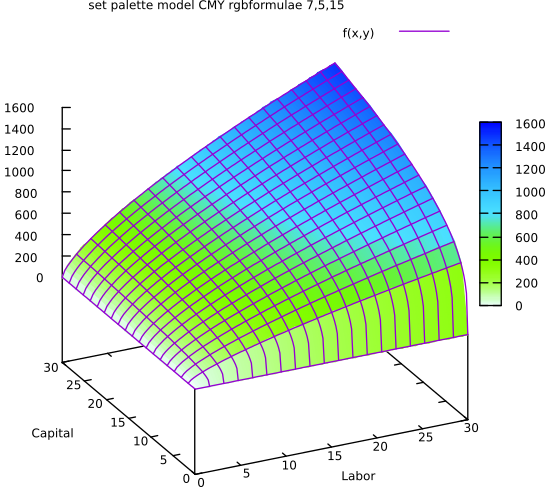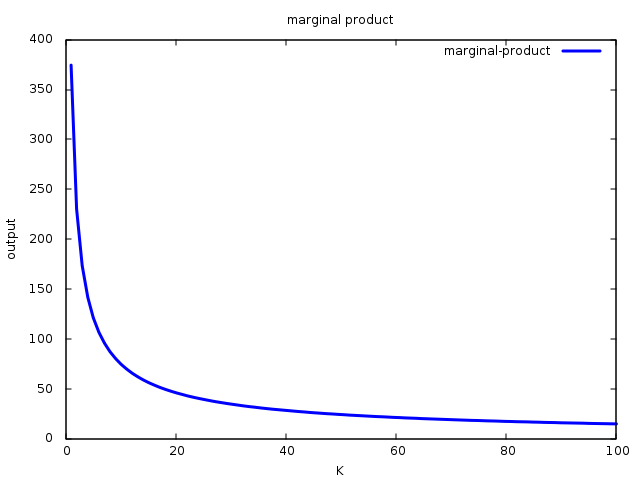### Site Tools

en:cobb-douglas-production-function

# Cobb-Douglas Production Function

The Cobb-Douglas Production Function is a particular form of the Production Function.

It takes the following form:

Q(L,K) = A Lβ Kα

• L:labor
• K:capital
• Q:output
• A>0
• 0<α<1
• 0<β<1

# Properties of Cobb Douglas Production Function

1. The marginal product is positive and decreasing.
2. Output elasticity is constant, equal to α for L or β for K.

Plot of a Cobb-Douglas production function:## The marginal product is positive and decreasing

This behavior is usually seen in a lot of real world examples. To find the marginal product of a production factor, we derivate the total output with respect that factor. For example, to find the marginal product of capital:

∂Q / ∂K =

= α * (A Lβ ) K(α-1)

Given that α is positive and lower than 1, the marginal product is positive and decreasing.

Graphically:## Output elasticity is constant

Output elasticity is defined as the percent change in output, when there is a percent change in one production factor.

In the case of the Cobb-Douglas production function, output elasticity is constant. Output elasticity of labor is β and output elasticity of capital is α.

### Proof

By definition, output elasticity is:

(∂Q/Q) / (∂L/L) =

= (∂Q/∂L) / (Q/L) That is the marginal product of labor divided the medium product of labor.

= [ Aβ Lβ-1 ) Kα ] / [ A Lβ Kα / L ]

Given that 1/L is L-1 , ALβ Kα /L is ALβ-1 Kα . It follows that output elasticity is:

= AβLβ-1 Kα / ALβ-1 Kα

The only difference between the numerator and the denominator is β; then:

Output elasticity = AβLβ-1 Kα / ALβ-1 Kα = β

Returns to scale measure the proportional change in output, given a proportional change in the quantity of every factor of production.

### Proof

If we increase every factor in a given constant c, the new output level will be:

Q' = A(cL)β (cK)α

= Acβ Lβ cα Kα

= cβ cα ALβ Kα

= cβ+α Q

This means that if we increase every production factor by c, the output level will increase in cβ+α .

If β+α = 1, the output will increase in c. In this case, the Cobb-Douglas production function has constant return to scale.

If β+α < 1, the proportional increase in output will be lower than the proportional increase in production factors. In this case, the Cobb-Douglas production function has decreasing returns to scale.

If β+α > 1, the proportional increase in output will be higher than the proportional increase in production factors. In this case, the production function has increasing returns to scale.

## Discussion

, 2017/12/23 13:42

If it is possible can someone post a real applicable example of how to calculate the elasticity of output with respect to labor and capital on Cobb Douglas function

, 2018/03/01 10:06, 2018/03/01 10:07

Example function:

 Q=10 L^0.4 K^0.6

Output elasticity (OE) with respect to K:

 (∂Q/∂K) / (Q/K)

 (∂Q/∂K) = 10 L^0.4 0.6 K^-0.4
 (Q/K) = 10 L^0.4 K^-0.4

Then, the output elasticity is: / (notice that almost all components of  and  all the same, so they cancel each other.

 Output Elasticity = 0.6

, 2019/08/21 10:30

Complete case example should be better.

Enter your comment. Wiki syntax is allowed:
P​ B U H A